# AP Biology : Cellular energetics

## Example Questions

← Previous 1

### Example Question #1 : Understand Atp Coupling

Given the hydrolysis of 1 mole of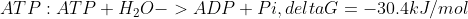, which reaction could be driven by hydrolyzing 2 moles of ATP?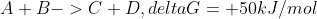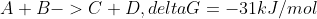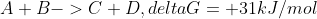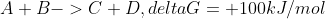Explanation:

Given that the hydrolysis of 1 mole of ATP has delta G of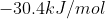, if 2 moles of ATP are hydrolyzed, delta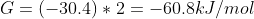. Reaction D has a delta G of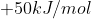; when coupled with the hydrolization of 2 moles of ATP, delta G will be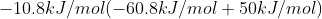. As this is negative, it is indicated that this will be a spontaneous reaction, or can proceed without input of energy.

### Example Question #2 : Understand Atp Coupling

ATP coupling describes the process through which hydrolysis of ATP can drive a __ reaction

thermodynamically favorable

spontaneous

thermodynamically unfavorable

exothermic

thermodynamically unfavorable

Explanation:

ATP coupling is the process where hydrolysis of ATP (a thermodynamically favorable, negative delta G, or spontaneous reaction) is coupled with a thermodynamically unfavorable reaction (a reaction that cannot proceed without energy input).

### Example Question #3 : Understand Atp Coupling

Which of the following is an endergonic reaction?

Hydrolysis of ATP

Catabolism

Na+/K+ pump

Cellular respiration

Na+/K+ pump

Explanation:

An endergonic reaction requires input of energy; delta G will be positive. Hydrolysis of ATP, cellular respiration, and catabolism (breakdown of a large molecule) are exergonic processes. The Na+/K+ pump is endergonic, as it requires energy. This process is coupled with ATP hydrolysis to allow it to proceed.

### Example Question #4 : Understand Atp Coupling

Which is true of an exergonic reaction?

The reaction will not occur spontaneously

Energy is required

The reaction will always be endothermic

Energy is released

Energy is released

Explanation:

Exergonic reactions are reactions that release energy. Endergonic reactions are reactions that require energy to proceed. Exergonic reactions can occur spontaneously, or are thermodynamically favorable. Endergonic reactions cannot occur spontaneously, or are thermodynamically unfavorable.

### Example Question #1 : Understand Atp Coupling

ATP phosphorylation (ADP+ Pi-> ATP+ H2O) is what type of reaction?

spontaneous

exergonic

endergonic

catabolic

endergonic

Explanation:

ATP hydrolysis is exergonic. ATP phosphorylation is the reverse of ATP hydrolysis, and thus is endergonic.

### Example Question #1 : Understand Atp Coupling

If the reaction for ATP hydrolysis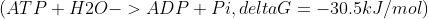is coupled with the reaction glucose+ sucrose-> fructose,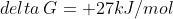, what is the net delta G?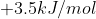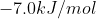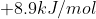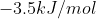Explanation:

Through ATP coupling, the hydrolysis of ATP can allow the second, thermodynamically unfavorable reaction to proceed.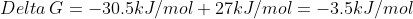. As the resulting delta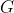is negative, this will be thermodynamically favorable.

### Example Question #1 : Understand Structure And Importance Of Atp

Which is NOT a reason that the phosphoanhydride bonds of ATP are high energy?

Resonance stabilization of products of ATP hydrolysis

Large mass of phosphate provides significant energy when combusted

Entropy is increased when bonds are broken

Repulsion of positively charged phosphate and negatively charged oxygen

Large mass of phosphate provides significant energy when combusted

Explanation:

The phosphoanhydride bonds of ATP, or the bonds between phosphate molecules, are high energy. This is due to the close proximity of positively charged phosphate and negatively charged oxygen; these charges repel. Also, there is resonance stabilization of the products of ATP hydrolysis (ADP and Pi); thus ADP is more stable than ATP. Additionally, entropy is increased when ATP is hydrolyzed; the second law of thermodynamics states that the entropy of a system always increases.

### Example Question #2 : Cellular Energetics

Which is not part of an ATP molecule?

threonine

alpha phosphate, beta phosphate, and gamma phosphate groups

ribose sugar

threonine

Explanation:

ATP consists of 3 phosphate groups, a ribose sugar, and adenine.

### Example Question #1 : Cellular Energetics

Which is true of an ATP molecule?

When hydrolyzed, a phosphoanhydride bond is formed

It is used for long-term energy storage

When hydrolyzed, delta G> 0

It is unstable

It is unstable

Explanation:

ATP is unstable; there is close proximity of positively charged phosphate and negatively charged oxygen; these charges repel. When hydrolyzed, delta G is <0 (exergonic). ATP is not used for long term-energy storage, as it is unstable. When hydrolyzed, a phosphoanhydride bond is broken.

### Example Question #3 : Cellular Energetics

ATP is produced when ADP is broken down

It contains 2 phosphate groups

It contains 3 phosphate groups

It contains more energy than ATP